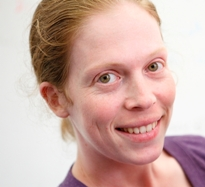# THEMATIC PROGRAMSJune  3, 2023Thematic Program on Asymptotic Geometric Analysis July - December 2010

## September 17,20-21, 2010 Fields Institute

 Thematic Home page Audio and Slides of talks Distinguished and Coxeter Lecturers
 Lecture 1: Abstract duality, the Legendre transform and a new duality transform. Defining abstract duality as an order reversing involution, the only such transform on the class of convex functions is the Legendre transform. Restricting, however, the class to non-negative convex functions which are pinned down to equal zero at the origin, an essentially different duality transform appears. We will describe it, prove uniqueness results, and point out geometric interpretations and consequences, for example concerning the support and the Minkowski functional for log-concave functions. Based on joint work with V. Milman Lecture 2: Order isomorphisms and the fundamental theorem of affine geometry. The fundamental theorem of affine geometry and its variants play a central role in determining order isomorphisms on classes of functions, as was also observed in the first lecture. We will discuss two other such examples. The first is that of convex functions on "windows", that is, whose domain is a convex set. The variant of the fundamental theorem is then of projective nature. We will see this is connected to the second duality discussed in lecture 1. The second example is order isomorhisms on vector spaces ordered by cones, where a new version of the fundamental theorem valid for a finite number of directions is needed. We will prove this theorem and its consequence for order isomorphisms. [Based on joint work with D. Florentin and V. Milman, and on joint work with B. Slomka] Lecture 3: Multiplicative transforms and characterization of the Fourier transform. The Fourier transform can be characterized as the unique transform (on a certain class) that exchanges product and convolution. We will discuss this result, together with related results concerning multiplicative transforms on certain classes of functions, and possibly also stability results for several functional equations. [Based on joint work with S. Alesker, D. Faifman and V. Milman]
Shiri Artstein-Avidan is a Senior Lecturer at the School of Mathematical Science at Tel Aviv University and received her Ph.D. from Tel Aviv University in 2004. Her thesis was titled 'Entropy Methods'.